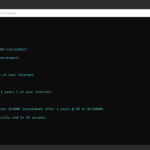## Compound Interest Calculator In C# With Source Code

Compound Interest Calculator in C# is based on the concept for the user convenience, to help them find out about the addition of interest to the principal sum of a loan or deposit. This project is for financial calculating to figure out the loan’s payment...

Type : Project

File Size : 299.6 KB# Similar Projects and Reports

#### Compound Interest Calculator In C# With Source Code

Compound Interest Calculator in C# is based on the concept for the user convenience, to help them find out about the addition of interest to the principal sum of a loan or deposit. This project is for financial calculating to figure out the loan’s payment...

#### Compound Interest Calculator In JavaScript With Source Code

Compound Interest Calculator is a simple project in HTML5, CSS, and JavaScript. This is an amazing calculator made for the user convenient, to help them find out about the addition of interest to the principal sum of a loan or deposit. This project is for...

#### Simple Interest Calculator In C++ With Source code

The Simple Interest Calculator is based on the concept of mathematical calculations of loan amount. This whole system is in the C++ language. The project is for user convenience, to help them find out about the monthly payments on a specific loan. This pr...

#### CALCULATOR IN C++ WITH SOURCE CODE

This project is based on a concept to generate the total sum, subtractions, divisions and multiplication. User has to input numbers with mathematical signs (“+,-,*,/”), the system will display the output. [ user has to input : 5*5, then the system will d...

#### Calculator App In C# With Source Code

This Calculator App in C# is based on the concept of normal mathematical operations on the calculator. The project contains the numbers, operators, and signs like in a normal calculator. Here, the user cannot enter the number themselves, they can just cli...

#### Simple Calculator In C# With Source Code

Simple Calculator in C# is based on the concept of normal mathematical operations on the calculator. The project contains the numbers, operators, and signs like in a normal calculator. So, the user cannot enter the number themselves, they can just click o...